# Matrices

Matrices are powerful operators in algebra. They represent operations on vector spaces and are used everywhere in signal processing to represent geometrical transforms and to solve series of equations. In a general form, a matrix is an NxM construct such as: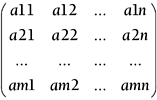Matrices can be added, multiplied, transposed, and inverted. The following sections contain some examples for 3x3 matrices.

Adding two matrices is as easy as adding their cells one by one, as shown in the following example: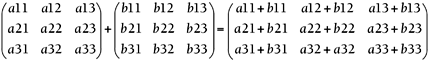Two matrices must be equally sized for addition to be possible.

#### Matrix Transposition

Some matrix and geometry operations require finding the transpose of a given matrix, which is defined as its mirror using the diagonal as the reflection axis, as shown here: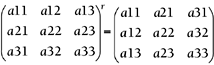#### Matrix-Matrix Multiplication

To multiply two matrices, their cell values must be multiplied using the following rule: To compute the cell at row X, column Y, we must perform the dot product of the X row from the first matrix with the Y column from the second matrix. Matrix multiplication is not commutative, so A*B is not necessarily equal to B*A. For a 3x3 case, here is the compact notation:So, for example, the element (1,2) from the result arises from taking the first row from A (hence, a1col, which means row 1, all columns) and multiplying it by the second column of B (brow2, meaning all rows from the second column of B).

A special case of matrix multiplication is applying a matrix to a vector, which is just a single-column matrix. Here is an example for a 3x3 matrix and corresponding vector: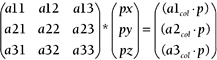#### Determinant of a Matrix

The determinant of a matrix is a numeric value that is computed from the matrix's cells. It is extremely important because other operations rely on this value. As you will soon see, the determinant is tightly coupled to the inverse of a matrix, which is very important for geometry. The determinant is only defined for square matrices and is represented by two vertical braces. For a general-sized matrix, it must be defined recursively. Here are the formulae for 2x2 and 3x3 cases: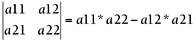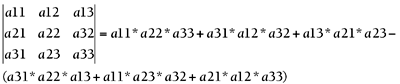#### Inverse of a Matrix

The inverse of a matrix A, represented by A-1, is a matrix such that A*A-1=Id, where Id is the identity matrix defined as:The inverse of a matrix is very useful for geometrical transforms. But not all matrices have inverses. A matrix A must have a nonzero determinant for the inverse to exist. In this case, the inverse is defined as follows: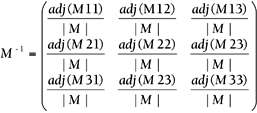where adj(Mij) denotes the adjoint for the element at row i, column j. The adjoint is the determinant of a special matrix that is constructed from M as follows: Take the submatrix from the original one so that the row and column denoted disappear, and the rest remains the same. Then, the signs for the newly created matrix must be swapped as follows: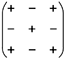For example, adj(M23) for a 3x3 matrix would be as follows: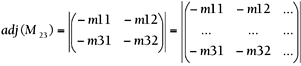#### Matrices for Geometry

Matrices are a compact and powerful tool used to describe geometrical transforms. In computer graphics and geometry in general the most popular form of matrix is the 4x4 homogeneous matrix, which is expressed as: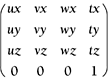The 3x3 top-left submatrix represents, in column order, the three basis vectors we want to implement. The fourth column labeled Tx, Ty, and Tz is the translate component. Given a point P, applying a transform simply means right-multiplying by the matrix while adding a trailing 1 to the point to add the translate component. Let's see this in detail: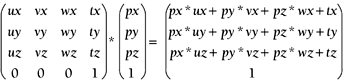Then, by specifying the different transforms by means of these matrices, we can apply geometric transforms such as rotations, scalings, shearings, and so on to the incoming vertices. Let's review some popular transforms and their associated matrices.

#### Translation

Translations can be easily expressed in terms of homogeneous matrices. The translation (Tx, Ty, Tz) is applied as follows: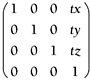Remember that translating is essentially adding. Thus, the neutral translation is translating by zero in X, Y, and Z.

#### Scaling

Scaling can be implemented in both homogeneous and nonhomogeneous terms. Whichever the case, the general scaling operation (Sx, Sy, Sz) is applied by a matrix like this: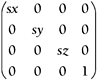Always remember that scaling is fundamentally a multiplication operation. Thus, the identity operation is not zero as in translation, but one. Scaling by (0,0,0) will collapse all your geometry, and quite likely generate some trouble in your graphics card.

#### Rotation

Rotation is a bit more complex than translating or scaling. It involves a bit more than adding or multiplying. We can define rotations around a canonical axis such as X (often called pitch), Y (often called yaw), and Z (often called roll). We can also define them around an arbitrary axis. We can also concatenate these matrices to perform compound rotations. Thus, there are different types of matrices we must become familiar with. To begin with, here are the roll, pitch, and yaw matrices:

Roll: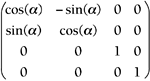Pitch: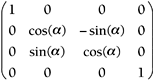Yaw: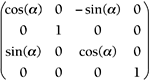#### Basis Matrices

A more general type of matrix can be used to define a basis function for an arbitrary coordinate system. Imagine that you have a character who is holding an item in his hand. In order to paint the item in place, you have two alternative options. First, you can describe its position and then determine its orientation by means of roll, pitch, and yaw matrices. Second, you can give the same position as in the first option, but supply the vectors that define the X, Y, and Z axis local coordinate system for the hand (see Figure D.2). This is especially useful for hierarchical animation. In a simpler case, if you are to paint a car in a rally game and the car must adapt to the terrain, you will not have the slope in terms of roll, pitch, and yaw. Quite likely, you will have the normal of the terrain and the direction the car is advancing toward.

##### Figure D.2. Local versus world coordinate systems.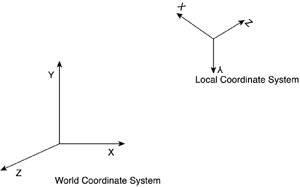The normal is the Y vector of the local coordinate system, and the advance direction takes the place of the Z vector. By computing the cross product between Y and Z (both converted to unit vectors), we will unveil an X vector, which will in fact emerge from the position of the driver and exit the car laterally, as shown in Figure D.3. Those three vectors are the basis that defines the local coordinate system for the car.

##### Figure D.3. Car showing the different coordinate systems.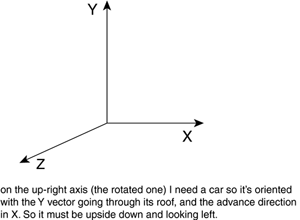Then, you can paint the car in its position and orientation by using basis matrices instead of simpler rotations and transforms. They are just plain homogeneous matrices that you will need to multiply all your vertices for. Given the three vectors defining the basis and the position vector, there are two important transform matrices.

The first matrix transforms from the world coordinate system into the object's coordinates. In other words, given a point above the car (in relative terms), such a matrix would allow us to know how high the point is from the car, even when the car is on a slope. The second matrix is the reverse process: Given a point in the car's coordinate system, it converts the point into world coordinates using the car's basis orientation matrix. For example, if we have a car in canonical orientation (Y meaning above and X meaning forward, for example) and want to place it in a point in space and in specific orientations, this is the matrix we would use.

For both matrices, we start with the basis of the space we want to move to. In this case, we create a 3x3 matrix whose columns are the new X, Y, and Z axes. Here is that matrix: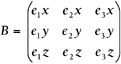So the basis is formed by the vectors e1, e2, and e3, representing the X, Y, and Z axes respectively.

Then, the reverse transform is defined as: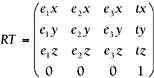where the top-left submatrix is B, and the rightmost vector is the translation from the old origin to the new one. This transform is usually called the rigid-body transform because it allows us to place a rigid body in space given the three orientation axes and a new origin.

The direct transform is then defined using the inverse we defined earlier. Specifically, it is defined as: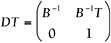Incidentally, if the basis B is orthonormal (column vectors are normalized, and they are mutually orthogonal), the inverse can be easily computed as, in this case, B-1=BT.

#### Concatenation of Transforms

The previous ideas can be extended by concatenating change-of-basis matrices so we can build hierarchical transforms. Imagine that we want to place a sword in the hand of a soldier who is in turn riding a horse. To do so, we would:

1. Transform to the horse's reference system

2. Transform to the rider's reference system

3. Transform to the hand

4. Place the sword

5. Detransform the sword

6. Detransform the hand

7. Detransform the rider

8. Detransform the horse

These are, respectively, reverse transforms (to reach the sword) and direct transforms (to get back). Thus, the composite transform would look something like this:

` Position(sword)=RT(horse)*RT(rider)*RT(hand)*Transform(sword)*DT(hand) *DT(rider)*DT(horse) `Core Techniques and Algorithms in Game Programming2003
ISBN: N/A
EAN: N/A
Year: 2004
Pages: 261